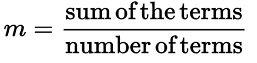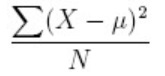# C program to calculate the mean, variance, and standard deviation of real numbers

Here, we are going to learn how to calculate the mean, variance, and standard deviation of real numbers using C program?
Submitted by Nidhi, on August 11, 2021

Problem Solution:

Create an array of real numbers and find the mean, variance, and standard deviation of numbers.

Mean of the real numbers:

The mean is the average of the numbers. It is easy to calculate the mean of the numbers: add up all the numbers, then divide by the total number of elements. To calculate the mean of the numbers, we use the formula,Variance of the real numbers:

The variance of the numbers is defined as: "The average of the squared differences from the Mean". To calculate the variance of the numbers, we use the formula,Standard deviation of the real numbers:

To find the standard deviation, we take the square root of the variance.

Program:

The source code to calculate the mean, variance and standard deviation of real numbers is given below. The given program is compiled and executed using GCC compile on UBUNTU 18.04 OS successfully.

```// C program to calculate the mean, variance, and
// standard deviation of real numbers

#include <stdio.h>
#include <math.h>

int main()
{
float arr = { 12.5, 14.5, 13.2, 8.95, 17.89 };

float sum;
float mean;
float variance;
float deviation;

int i = 0;

for (i = 0; i < 5; i++)
sum = sum + arr[i];

mean = sum / 5;

sum = 0;
for (i = 0; i < 5; i++) {
sum = sum + pow((arr[i] - mean), 2);
}
variance = sum / 5;

deviation = sqrt(variance);

printf("Mean of elements    : %.2f\n", mean);
printf("variance of elements: %.2f\n", variance);
printf("Standard deviation  : %.2f\n", deviation);

return 0;
}
```

Output:

```Mean of elements    : 13.41
variance of elements: 8.40
Standard deviation  : 2.90
```

Explanation:

Here, we created an array arr of floating-point numbers with 5 elements. Then we calculated the mean, variance, and standard deviation of array elements. After that, we printed the result on the console screen.